## Calculating Pascal’s Triangle in SQL Server

Mar 15

Written by: Charles Flock
3/15/2011 7:07 PMWe demonstrate the kind of things that you can do in SQL Server using the XLeratorDB function library. Would you ever really need to build Pascal’s triangle in T-SQL? Probably not. But you really don’t need to build it in any programming language, yet when I search on ‘programming Pascal’s Triangle’ in Google I get about 139,000 results. Now there’s one more.

Don’t worry if you don’t remember Pascal’s Triangle from high school math class. This is what wikipedia is for. Here’s a little visual representation that might jog your memory.
1
1 1
1 2 1
1 3 3 1
1 4 6 4 1
In this article, I will show you how to create Pascal’s Triangle using T-SQL and a few functions from the XLeratorDB function library. In order to do that, let’s turn the triangle into a right triangle instead of an isosceles triangle.

 1 1 1 1 2 1 1 3 3 1 1 4 6 4 1

This looks like something that has rows and columns, so we should be able use T-SQL to create the triangle. Notice, though, that there are a different number of columns for each row. In fact, the number of columns is equal to the row number. The first row has one column and the fifth row has five columns. Also notice that the first column always has a value of 1. Both of these attributes need to be incorporated in the T-SQL. You will also notice that when the column number is equal to the row number, the column value always has a value of 1.
There a number of ways to calculate the values in the other columns. Mathematically, though, each column (c) in each row (r) is calculated as: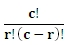This equation is immediately recognizable as the equation for calculating the number of combinations. XLeratorDB provides this capability in the COMBIN function, which works exactly the same way as the EXCEL COMBIN function. We can use this function to calculate the value in each column, except the first column where the value will always be 1. All we need to do is create the appropriate row and column information and we can calculate the value for each node.
We can use the XLeratorDB SeriesInt function to sequentially generate numbers for the number of rows and the number of columns. In this statement will put all the pieces together to create the values for a triangle with 5 rows.
SELECT r,
c,
CASE
WHEN r = c then 1
ELSE wct.COMBIN(r, c)
END   as v
FROM (
SELECT m.Seriesvalue as r, k.SeriesValue as c
FROM wct.SeriesInt(0, 4, NULL, NULL, NULL) m
,wct.SeriesInt(0, 4, NULL, NULL, NULL) k
) n
WHERE c <= r
ORDER BY r, c
This produces the following result.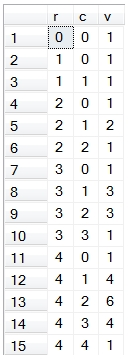This gives us all the correct values, but it doesn’t look much like a triangle. We can try to use the PIVOT function to make it look like a triangle.

SELECT ,,,,,
FROM (
SELECT
r,
c,
CASE
WHEN r = c then 1
ELSE wct.COMBIN(r, c)
END   as v
FROM (
SELECT m.Seriesvalue as r, k.SeriesValue as c
FROM wct.SeriesInt(0, 4, NULL, NULL, NULL) m
,wct.SeriesInt(0, 4, NULL, NULL, NULL) k
) n
WHERE c <= r
) d
PIVOT(sum(v) for c in(,,,,,)
) as P

This produces the following result.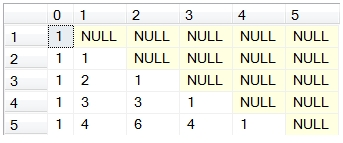This is better, but there a couple of problems. First, we haven’t really created a triangle; we have created a square. Sure, the diagonal of ones, where r = c, marks a lower diagonal which corresponds to Pascal’s triangle and the upper diagonal just consists of NULL, but it’s still a square and not a triangle.
The second problem is that we have to explicitly label each column (, , , , ,  in this case) in the both the PIVOT and the SELECT to get what we want. If we want to create the triangle for some other number of rows, we have to change a bunch of SQL. If we were writing a stored procedure, we could do this dynamically, but we are trying to do this in T-SQL. What we are looking for, then, is a way to join all the items in a row together and also avoid having to explicitly label each column. In other words, we are looking for a way to take the data in normal form and turn it into Pascal’s Triangle.
We can use the XLeratorDB JOINSTR_q function to create the triangle. In this example, we will modify our SQL slightly to accept a value which determines the number of rows we wish to create in the triangle and we will use the JOINSTR_q function to concatenate the values (in normal form) and create the triangle.

DECLARE @lvl as int = 4
SELECT r,
c,
CASE
WHEN r = c then 1
ELSE wct.COMBIN(r, c)
END   as v
into #p
FROM (
SELECT m.Seriesvalue as r, k.SeriesValue as c
FROM wct.SeriesInt(0, @lvl, NULL, NULL, NULL) m
,wct.SeriesInt(0, @lvl, NULL, NULL, NULL) k
) n
WHERE c <= r
SELECT wct.JOINSTR_q(' ',NULL,'SELECT v from #p where r = ' + cast(x.r as varchar) + ' ORDER BY c')
FROM #p x
GROUP by x.r

DROP TABLE #p

This produces the following result.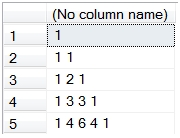This looks exactly like the triangle in our example. We have created a very simple piece of T-SQL which lets us specify any level of the triangle and automatically returns the results in triangle form. In this example, we will go to 10 levels and create the triangle using comma, rather than space, as a separator.

DECLARE @lvl as int = 10
SELECT r,
c,
CASE
WHEN r = c then 1
ELSE wct.COMBIN(r, c)
END   as v
into #p
FROM (
SELECT m.Seriesvalue as r, k.SeriesValue as c
FROM wct.SeriesInt(0, @lvl, NULL, NULL, NULL) m
,wct.SeriesInt(0, @lvl, NULL, NULL, NULL) k
) n
WHERE c <= r
SELECT wct.JOINSTR_q(',',NULL,'SELECT v from #p where r = ' + cast(x.r as varchar) + ' ORDER BY c')
FROM #p x
GROUP by x.r

DROP TABLE #p

This produces the following result.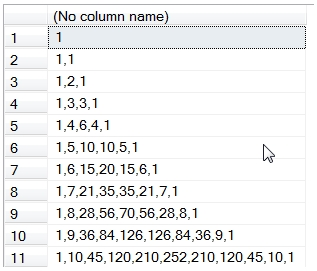As you can see, what could otherwise be a very complicated task in T-SQL, is greatly simplified by having a couple of very familiar functions available to us in the database. In fact, the logic in this example is almost exactly the same as the logic you would use in spreadsheet, which might look something like this.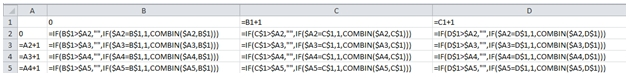It actually seems easier to create Pacal’s Triangle in T-SQL than it would be in EXCEL. There’s no EXCEL function to concatenate the results into a triangle, and EXEL suffers from the same problem as PIVOT, in that it creates a square and not a triangle. What do you think?

Tags:
Categories:
Location: BlogsThe WestClinTech Blog

## Search Blogs

 KeywordsPhrase

## Blog Archives

Archive
 < December 2023 >
SunMonTueWedThuFriSat
262728293012
3456789
10111213141516
17181920212223
24252627282930
31123456
Monthly
Go

### SupportCopyright 2008-2023 Westclintech LLC         Privacy Policy        Terms of Service